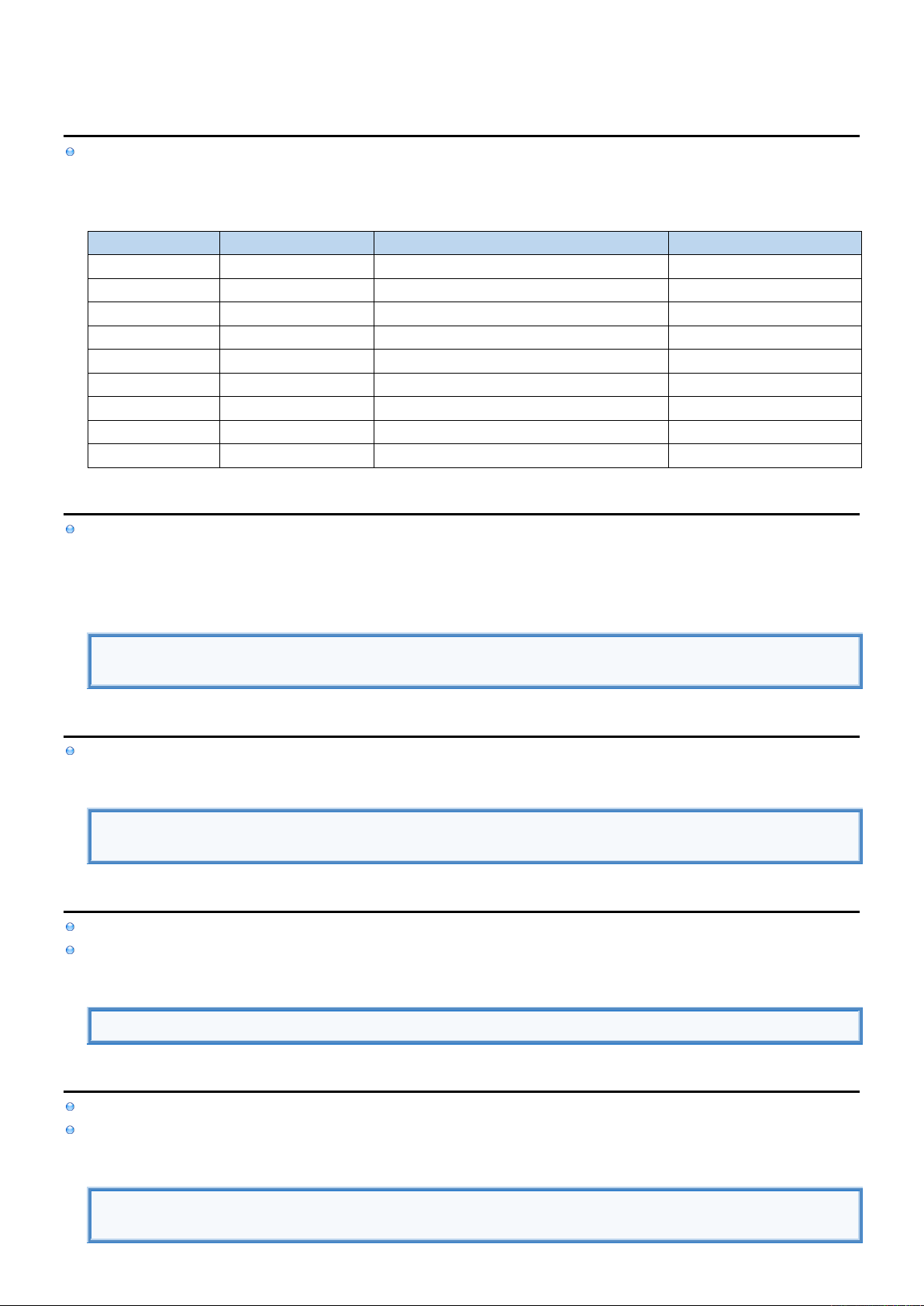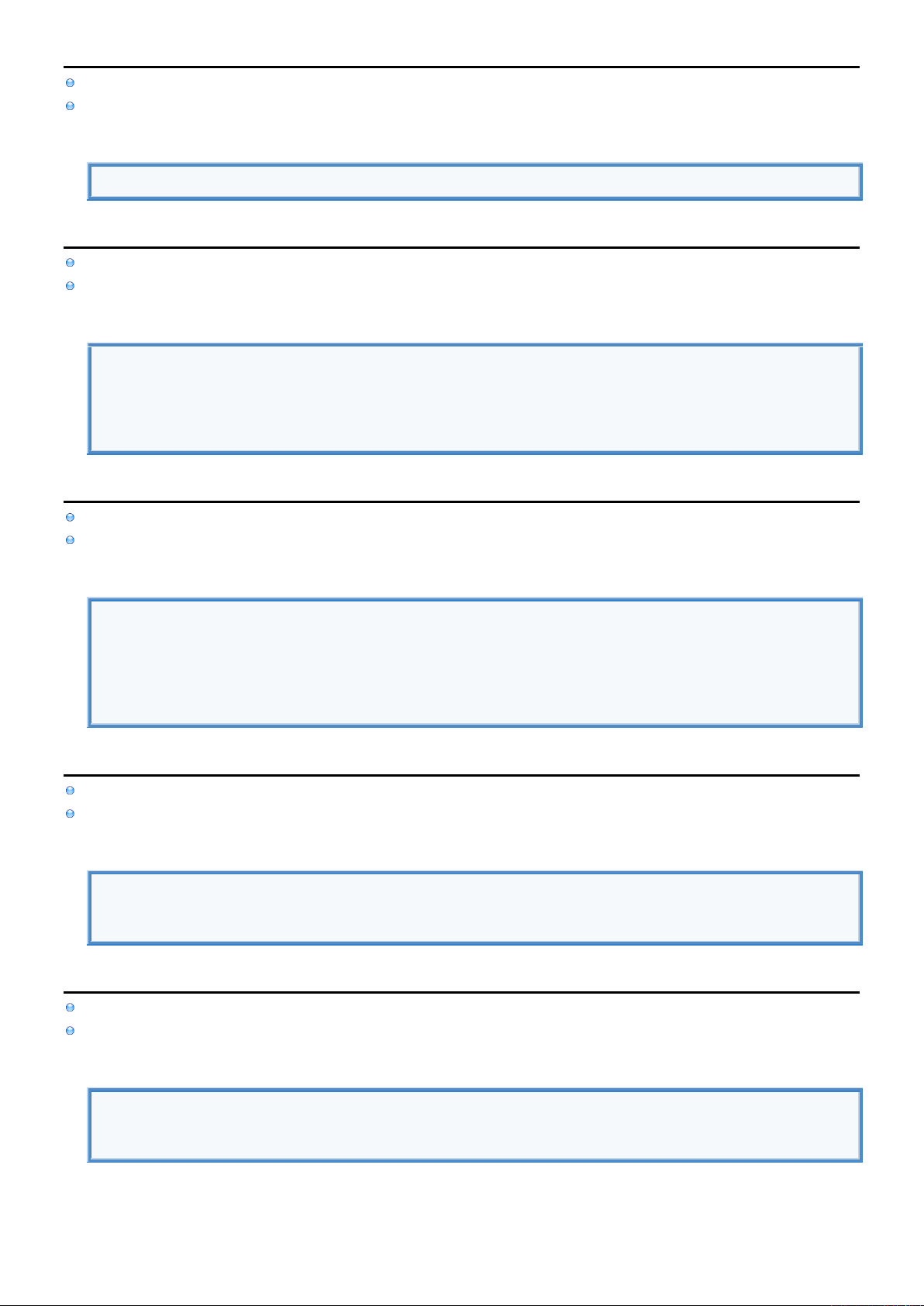2
2
.
.
1
1
.
.
2
2
S
S
c
c
a
a
l
l
a
a
r
r
s
s
I
I
n
n
f
f
o
o
[
[
R
R
]
]
Scalar Data Types can only store single value. In Kotlin every Data Type is an Object.
Unlike Java which has primitive Data Types (int, double) and related wrapper Objects (Integer, Double).
Scalar Data Types
TYPE
EXAMPLE
DESCRIPTION
SIZE
Null
null
undefined/unknown value
Bool
true, false
logical TRUE or FALSE
Char
Unicode character
16-bit
Byte
20, -54
Signed integer number
8-bit two's complement
Short
20, -54
Signed integer number
16-bit two's complement
Int
20, -54
Signed integer number
32-bit two's complement
Long
20, -54
Signed integer number
64-bit two's complement
Float
-45.87
Real number
32-bit IEEE 754
Double
-45.87
Real number
64-bit IEEE 754
N
N
u
u
l
l
l
l
Null Data Type can only have null value and can only be assigned to Optional either by
explicitly setting Optional to null using Null Literal
declaring Optional without assigning value to it in which case it is initialized to null
Null
var age : Int? //Optional variable is initialized to null data type.
var weight : Int? = null //Optional variable is set to null data type using Null Literal.
B
B
o
o
o
o
l
l
e
e
a
a
n
n
Bool Data Type can only have two possible values logical true or false and can be created using Boolean Literal.
Boolean
var access2 : Boolean = false //Explicit Data Type declaration.
var access1 = true //Implicit Data Type declaration.
C
C
h
h
a
a
r
r
Float data type is type of data that represents real number and can be created using Float Literal.
Check out float cheat sheet containing code samples for working with float data type.
Char
var value : Char = 'A'
B
B
y
y
t
t
e
e
Byte data type is type of data that represents integer number and can be created using Integer Literal.
Check out byte cheat sheet containing code samples for working with byte data type.
byte
var value: Byte = 65 //Create byte data using Integer Literal which is then implicitly converted to byte
data.S
S
h
h
o
o
r
r
t
t
Short data type is type of data that represents integer number and can be created using Integer Literal.
Check out short cheat sheet containing code samples for working with short data type.
Short
var value: Short = 65 //Create short data using integer literal which is implicitly converted to short data.
I
I
n
n
t
t
Int data type is type of data that represents integer number and can be created using Integer Literal.
Check out int cheat sheet containing code samples for working with int data type.
Int
//CREATE INTEGER DATA USING INTEGER LITERAL IN DIFFERENT NOTATIONS.
var value : Int = 65 //Decimal.
value = 0b1000001 //Binary. 0B1000001
value = 10_000 //You can use underscore any way you like: 10_000, 0b10__00_001
L
L
o
o
n
n
g
g
Long data type is type of data that represents integer number and can be created using Long Literal.
Check out long cheat sheet containing code samples for working with long data type.
Long
//CREATE LONG DATA USING INTEGER LITERAL IN DIFFERENT NOTATIONS.
var longValue = 1000L //Use "L" sufix to specify Long value when Data Type is not declared
var value : Long = 65 //Decimal.
value = 0b1000001 //Binary. 0B1000001
value = 10_000 //You can use underscore any way you like: 10_000, 0b10__00_001
F
F
l
l
o
o
a
a
t
t
Float Data Type is type of data that represents real number and can be created using Float Literal.
Check out float cheat sheet containing code samples for working with float data type.
Float
//CREATE FLOAT DATA USING FLOAT LITERAL IN DIFFERENT NOTATIONS.
var value : Float = 65.23f //Basic notation. 65F
value = 0.6523E2f //Scientific notation. 0.6523E2 F
D
D
o
o
u
u
b
b
l
l
e
e
Double data type is type of data that represents real number and can be created using Double Literal.
Check out double cheat sheet containing code samples for working with double data type.
Double
//CREATE DOUBLE DATA USING DOUBLE LITERAL IN DIFFERENT NOTATIONS.
var value : Double = 65.23 //Basic notation. 65F
value = 0.6523E2 //Scientific notation. 0.6523E2 F